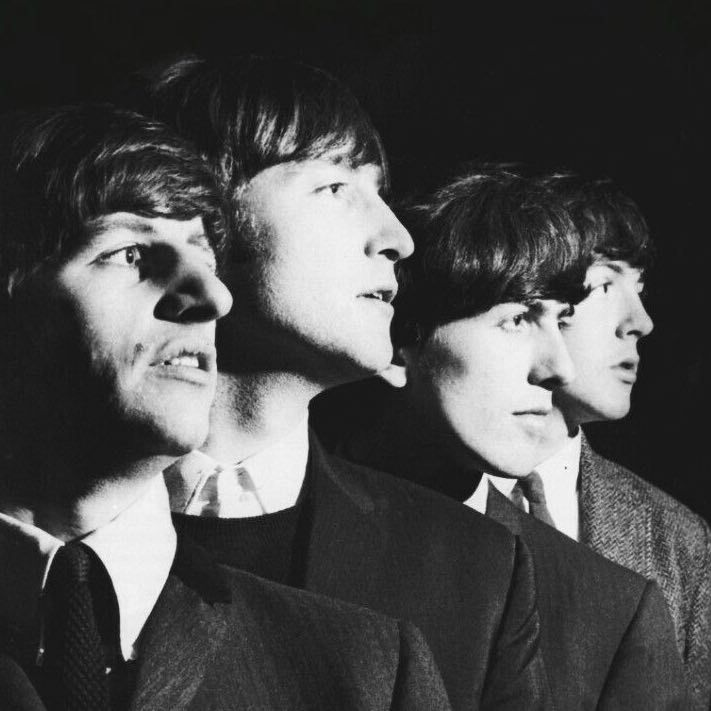# Chord Gitar The Beatles - Let it Be### + The Beatles - Let it Be +

When I find myself in times of trouble Mother Mary comes to me Speaking words of wisdom Let it be And in my hour of darkness She is standing right in front of me Speaking words of wisdom Let it be Let it be, let it be, let it be, let it be Whisper words of wisdom Let it be And when the broken-hearted people Living in the world agree There will be an answer Let it be For though they may be parted there is Still a chance that they will see There will be an answer Let it be Let it be, let it be, let it be, let it be Yeah, there will be an answer Let it be Let it be, let it be, let it be, let it be Whisper words of wisdom Let it be Let it be, let it be, let it be, let it be Whisper words of wisdom Let it be And when the night is cloudy There is still a light that shines on me Shine until tomorrow Let it be I wake up to the sound of music Mother Mary comes to me Speaking words of wisdom Let it be Let it be, let it be, let it be, yeah, let it be There will be an answer Let it be Let it be, let it be, let it be, yeah, let it be There will be an answer Let it be Let it be, let it be, let it be, yeah, let it be Whisper words of wisdom Let it be
```Intro :
C G Am F
C G F Em Dm C

C              G
when I find myself in times of trouble
Am          F
mother mary comes to me
C                G
speaking words of wisdom
F  Em Dm C
let it be..

C                 G
and in my hour of darkness
Am               F
she is standing right in front of me
C                 G
speaking words of wisdom
F  Em Dm C
let it be..

Reff :
Am          G
let it be.. let it be..
F           C
let it be.. let it be..
C                G
whisper words of wisdom
F  Em Dm C
let it be..

C               G
and when the broken hearted people
Am            F
living in the world agree
C                G
F  Em Dm C
let it be..

C                  G
for though they may be parted
Am                  F
there is still a chance that they will see
C                  G
F  Em Dm C
let it be..

Reff :
Am          G
let it be.. let it be..
F           C
let it be.. let it be..
C                G
ya there will be an answer
F  Em Dm C
let it be..

Am          G
let it be.. let it be..
F           C
let it be.. let it be..
C                G
whisper words of wisdom
F  Em Dm C
let it be..

Musik :
F  Em Dm C Bb Am G F C
F C G  F C
C G Am F
C G F  Em Dm C
C G Am F
C G F  Em Dm C

Reff :
Am          G
let it be.. let it be..
F              C
let it be ye.. let it be..
C                G
whisper words of wisdom
F  Em Dm C
let it be..

C                 G
and when the night is cloudy
Am                 F
there«is still a light that shines on me
C             G
shine until tomorrow
F  Em Dm C
let it be..

C              G
I wake up to the sound of music
Am          F
mother mary comes to me
C                 G
speaking words of wisdom
F  Em Dm C
let it be.. he yeach..

Reff :
Am          G
let it be.. let it be..
F              C
let it be ye.. let it be..
C                G
oh there will be an answer
F  Em Dm C
let it be.. he..

Am          G
let it be.. let it be..
F              C
let it be ye.. let it be..
C                G
oh there will be an answer
F  Em Dm C
let it be.. he..

Am          G
let it be.. let it be..
F              C
let it be ye.. let it be..
C                G
whisper words of wisdom
F  Em Dm C
let it be..

Outro :
F Em Dm C Bb Am G F C```